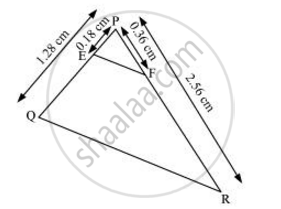# E and F are points on the sides PQ and PR respectively of a ΔPQR. For the following case, state whether EF || QR. PQ = 1.28 cm, PR = 2.56 cm, PE = 0.18 cm and PF = 0.36 cm - Mathematics

E and F are points on the sides PQ and PR respectively of a ΔPQR. For the following case, state whether EF || QR. PQ = 1.28 cm, PR = 2.56 cm, PE = 0.18 cm and PF = 0.36 cm

#### SolutionPQ = 1.28 cm, PR = 2.56 cm, PE = 0.18 cm, PF = 0.36 cm

(PE)/(PQ) = 0.18/1.28 = 18/128=9/64

(PF)/(PR) = 0.36/2.56=9/64

Hence (PE)/(PQ) = (PF)/(PR)

Therefore EF is parallel to QR

Concept: Similarity of Triangles
Is there an error in this question or solution?

#### APPEARS IN

NCERT Class 10 Maths
Chapter 6 Triangles
Exercise 6.2 | Q 2.3 | Page 128